# Cyclical Learning Rates with Keras and Deep Learning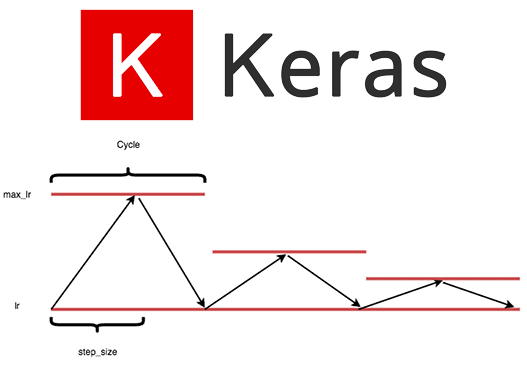In this tutorial, you will learn how to use Cyclical Learning Rates (CLR) and Keras to train your own neural networks. Using Cyclical Learning Rates you can dramatically reduce the number of experiments required to tune and find an optimal learning rate for your model.

Today is part two in our three-part series on tuning learning rates for deep neural networks:

1. Part #1: Keras learning rate schedules and decay (last week’s post)
2. Part #2: Cyclical Learning Rates with Keras and Deep Learning (today’s post)
3. Part #3: Automatically finding optimal learning rates (next week’s post)

Last week we discussed the concept of learning rate schedules and how we can decay and decrease our learning rate over time according to a set function (i.e., linear, polynomial, or step decrease).

However, there are two problems with basic learning rate schedules:

1. We don’t know what the optimal initial learning rate is.
2. Monotonically decreasing our learning rate may lead to our network getting “stuck” in plateaus of the loss landscape.

Cyclical Learning Rates take a different approach. Using CLRs, we now:

1. Define a minimum learning rate
2. Define a maximum learning rate
3. Allow the learning rate to cyclically oscillate between the two bounds

In practice, using Cyclical Learning Rates leads to faster convergence and with fewer experiments/hyperparameter updates.

And when we combine CLRs with next week’s technique on automatically finding optimal learning rates, you may never need to tune your learning rates again! (or at least run far fewer experiments to tune them).

To learn how to use Cyclical Learning Rates with Keras, just keep reading!

Looking for the source code to this post?

## Cyclical Learning Rates with Keras and Deep Learning

In the first part of this tutorial, we’ll discuss Cyclical Learning Rates, including:

• What are Cyclical Learning Rates?
• Why should we use Cyclical Learning Rates?
• How do we use Cyclical Learning Rates with Keras?

From there, we’ll implement CLRs and train a variation of GoogLeNet on the CIFAR-10 dataset — I’ll even point out how to use Cyclical Learning Rates with your own custom datasets.

Finally, we’ll review the results of our experiments and you’ll see firsthand how CLRs can reduce the number of learning rate trials you need to perform to find an optimal learning rate range.

### What are cyclical learning rates?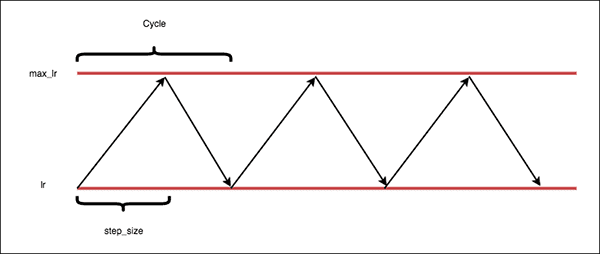Figure 1: Cyclical learning rates oscillate back and forth between two bounds when training, slowly increasing the learning rate after every batch update. To implement cyclical learning rates with Keras, you simply need a callback.

As we discussed in last week’s post, we can define learning rate schedules that monotonically decrease our learning rate after each epoch.

By decreasing our learning rate over time we can allow our model to (ideally) descend into lower areas of the loss landscape.

In practice; however, there are a few problems with a monotonically decreasing learning rate:

• First, our model and optimizer are still sensitive to our initial choice in learning rate.
• Second, we don’t know what the initial learning rate should be — we may need to perform 10s to 100s of experiments just to find our initial learning rate.
• Finally, there is no guarantee that our model will descend into areas of low loss when lowering the learning rate.

To address these issues, Leslie Smith of the NRL introduced Cyclical Learning Rates in his 2015 paper, Cyclical Learning Rates for Training Neural Networks.

1. Define the lower bound on our learning rate (called “base_lr”).
2. Define the upper bound on the learning rate (called the “max_lr”).
3. Allow the learning rate to oscillate back and forth between these two bounds when training, slowly increasing and decreasing the learning rate after every batch update.

An example of a Cyclical Learning Rate can be seen in Figure 1.

Notice how our learning rate follows a triangular pattern. First, the learning rate is very small. Then, over time, the learning rate continues to grow until it hits the maximum value. The learning rate then descends back down to the base value. This cyclical pattern continues throughout training.

### Why should we use Cyclical Learning Rates?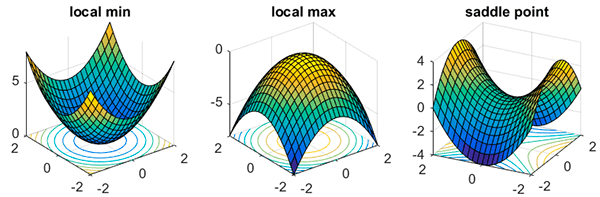Figure 2: Monotonically decreasing learning rates could lead to a model that is stuck in saddle points or a local minima. By oscillating learning rates cyclically, we have more freedom in our initial learning rate, can break out of saddle points and local minima, and reduce learning rate tuning experimentation. (image source)

As mentioned above, Cyclical Learning Rates enables our learning rate to oscillate back and forth between a lower and upper bound.

So, why bother going through all the trouble?

Why not just monotonically decrease our learning rate, just as we’ve always done?

The first reason is that our network may become stuck in either saddle points or local minima, and the low learning rate may not be sufficient to break out of the area and descend into areas of the loss landscape with lower loss.

Secondly, our model and optimizer may be very sensitive to our initial learning rate choice. If we make a poor initial choice in learning rate, our model may be stuck from the very start.

Instead, we can use Cyclical Learning Rates to oscillate our learning rate between upper and lower bounds, enabling us to:

1. Have more freedom in our initial learning rate choices.
2. Break out of saddle points and local minima.

In practice, using CLRs leads to far fewer learning rate tuning experiments along with near identical accuracy to exhaustive hyperparameter tuning.

### How do we use Cyclical Learning Rates?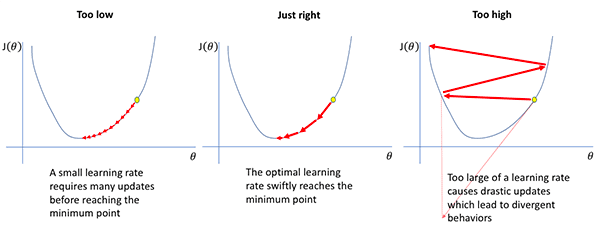Figure 3: Brad Kenstler’s implementation of deep learning Cyclical Learning Rates for Keras includes three modes — “triangular”, “triangular2”, and “exp_range”. Cyclical learning rates seek to handle training issues when your learning rate is too high or too low shown in this figure. (image source)

We’ll be using Brad Kenstler’s implementation of Cyclical Learning Rates for Keras.

In order to use this implementation we need to define a few values first:

• Batch size: Number of training examples to use in a single forward and backward pass of the network during training.
• Batch/Iteration: Number of weight updates per epoch (i.e., # of total training examples divided by the batch size).
• Cycle: Number of iterations it takes for our learning rate to go from the lower bound, ascend to the upper bound, and then descend back to the lower bound again.
• Step size: Number of iterations in a half cycle. Leslie Smith, the creator of CLRs, recommends that the step_size should be (2-8) * training_iterations_in_epoch). In practice, I have found that step sizes or either 4 or 8 work well in most situations.

With these terms defined, let’s see how they work together to define a Cyclical Learning Rate policy.

#### The “triangular” policyFigure 4: The “triangular” policy mode for deep learning cyclical learning rates with Keras.

The “triangular” Cyclical Learning Rate policy is a simple triangular cycle.

Our learning rate starts off at the base value and then starts to increase.

We reach the maximum learning rate value halfway through the cycle (i.e., the step size, or number of iterations in a half cycle). Once the maximum learning rate is hit, we then decrease the learning rate back to the base value. Again, it takes a half cycle to return to the base learning rate.

This entire process repeats (i.e., cyclical) until training is terminated.

#### The “triangular2” policy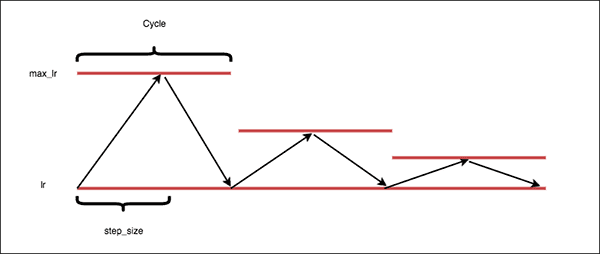Figure 5: The deep learning cyclical learning rate “triangular2” policy mode is similar to “triangular” but cuts the max learning rate bound in half after every cycle.

The “triangular2” CLR policy is similar to the standard “triangular” policy, but instead cuts our max learning rate bound in half after every cycle.

We can oscillate our learning rate to break out of saddle points/local minima…

…and at the same time decrease our learning rate, enabling us to descend into lower loss areas of the loss landscape.

Furthermore, reducing our maximum learning rate over time helps stabilize our training. Later epochs with the “triangular” policy may exhibit large jumps in both loss and accuracy — the “triangular2” policy will help stabilize these jumps.

#### The “exp_range” policy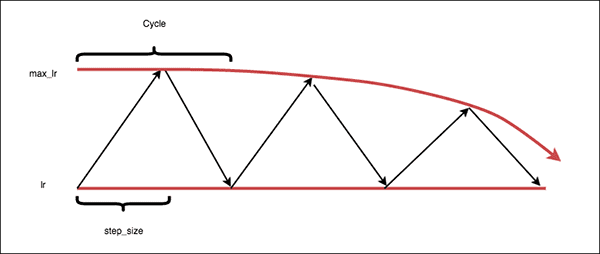Figure 6: The “exp_range” cyclical learning rate policy undergoes exponential decay for the max learning rate bound while still exhibiting the “triangular” policy characteristics.

The “exp_range” Cyclical Learning Rate policy is similar to the “triangular2” policy, but, as the name suggests, instead follows an exponential decay, giving you more fine-tuned control in the rate of decline in max learning rate.

Note: In practice, I don’t use the “exp_range” policy — the “triangular” and “triangular2” policies are more than sufficient in the vast majority of projects.

### How do I install Cyclical Learning Rates on my system?

The Cyclical Learning Rate implementation we are using is not pip-installable.

1. Use the “Downloads” section to grab the file and associated code/data for this tutorial.

From there, let’s move on to training our first CNN using a Cyclical Learning Rate.

### Project structure

Go ahead and run the tree  command from within the keras-cyclical-learning-rates/  directory to print our project structure:

The output/  directory will contain our CLR and accuracy/loss plots.

The pyimagesearch  module contains our cyclical learning rate callback class, MiniGoogLeNet CNN, and configuration file:

• The clr_callback.py  file contains the Cyclical Learning Rate callback which will update our learning rate automatically at the end of each batch update.
• Our config.py  is simply a Python file containing configuration variables — we’ll review it in the next section.

Our training script, train_cifar10.py , trains MiniGoogLeNet using the CIFAR-10 dataset. The training script takes advantage of our CLR callback and configuration.

### Our configuration file

Before we implement our training script, let’s first review our configuration file:

We will use the os  module in our config so that we can construct operating system-agnostic paths directly (Line 2).

From there, our CIFAR-10 CLASSES  are defined (Lines 5 and 6).

Let’s define our cyclical learning rate parameters:

The MIN_LR and MAX_LR define our base learning rate and maximum learning rate, respectively (Lines 10 and 11). I know these learning rates will work well when training MiniGoogLeNet per the experiments I have already run for Deep Learning for Computer Vision with Python — next week I will show you how to automatically find these values.

The BATCH_SIZE (Line 12) is the number of training examples per batch update.

We then have the STEP_SIZE which is the number of batch updates in a half cycle (Line 13).

The CLR_METHOD controls our Cyclical Learning Rate policy (Line 14). Here we are using the triangular policy, as discussed in the previous section.

We can calculate the number of full CLR cycles in a given number of epochs via:

NUM_CLR_CYCLES = NUM_EPOCHS / STEP_SIZE / 2

For example, with NUM_EPOCHS = 96 and STEP_SIZE = 8, there will be a total of 6 full cycles: 96 / 8 / 2 = 6.

Finally, we define our output plot paths/filenames:

We’ll plot a training history accuracy/loss plot as well as a cyclical learning rate plot. You may specify the paths + filenames of the plots on Lines 19 and 20.

### Implementing our Cyclical Learning Rate training script

With our configuration defined, we can move on to implementing our training script.

Open up trian_cifar10.py and insert the following code:

Lines 2-15 import our necessary packages. Most notably our CyclicLR  (from the clr_callback  file) is imported via Line 7. The matplotlib  backend is set on Line 3 so that our plots can be written to disk at the end of the training process.

Next, let’s load our CIFAR-10 data:

Lines 20-22 load the CIFAR-10 image dataset. The data is pre-split into training and testing sets.

From there, we calculate the mean  and apply mean subtraction (Lines 25-27). Mean subtraction is a normalization/scaling technique that results in improved model accuracy. For more details, please refer to the Practitioner Bundle of Deep Learning for Computer Vision with Python.

Labels are then binarized (Lines 30-32).

Next, we initialize our data augmentation object (Lines 35-37). Data augmentation increases model generalization by producing randomly mutated images from your dataset during training. I’ve written about data augmentation in-depth in Deep Learning for Computer Vision with Python as well as two blog posts (How to use Keras fit and fit_generator (a hands-on tutorial) and Keras ImageDataGenerator and Data Augmentation).

Let’s initialize (1) our model, and (2) our cyclical learning rate callback:

Our model  is initialized with stochastic gradient descent ( SGD ) optimization and "categorical_crossentropy"  loss (Lines 41-44). If you have only two classes in your dataset, be sure to set loss="binary_crossentropy" .

Next, we initialize the cyclical learning rate callback via Lines 48-52. The CLR parameters are provided to the constructor. Now is a great time to review them at the top of the “How do we use Cyclical Learning Rates?” section above. The step_size  follow’s Leslie Smith’s recommendation of setting it to be a multiple of the number of batch updates per epoch.

Let’s train and evaluate our model using CLR now:

Lines 56-62 launch training using the clr  callback and data augmentation.

Then Lines 66-68 evaluate the network on the testing set and print a classification_report .

Finally, we’ll generate two plots:

Two plots are generated:

• Training accuracy/loss history (Lines 71-82). The standard plot format included in most of my tutorials and every experiment of my deep learning book.
• Learning rate history (Lines 86-91). This plot will help us to visually verify that our learning rate is oscillating according to our intentions.

### Training with cyclical learning rates

We are now ready to train our CNN using Cyclical Learning Rates with Keras!

Make sure you’ve used the “Downloads” section of this post to download the source code — from there, open up a terminal and execute the following command:

As you can see, by using the “triangular” CLR policy we are obtaining 92% accuracy on our CIFAR-10 testing set.

The following figure shows the learning rate plot, demonstrating how it cyclically starts at our lower learning rate bound, increases to the maximum value at half a cycle, and then decreases again to the lower bound, completing the cycle: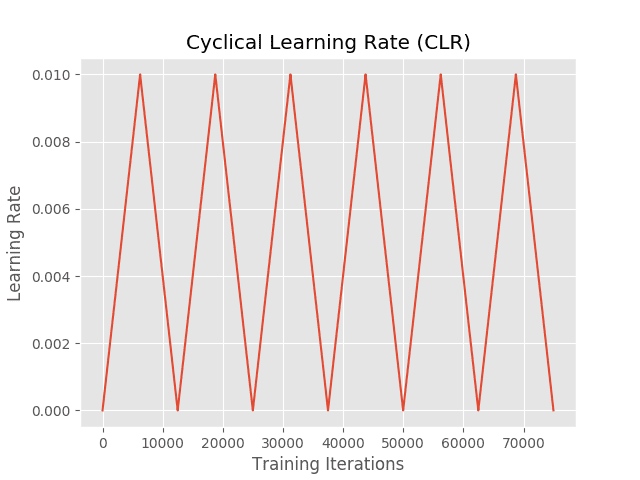Figure 7: Our first experiment of deep learning cyclical learning rates with Keras uses the “triangular” policy.

Examining our training history you can see the cyclical behavior of the learning rate: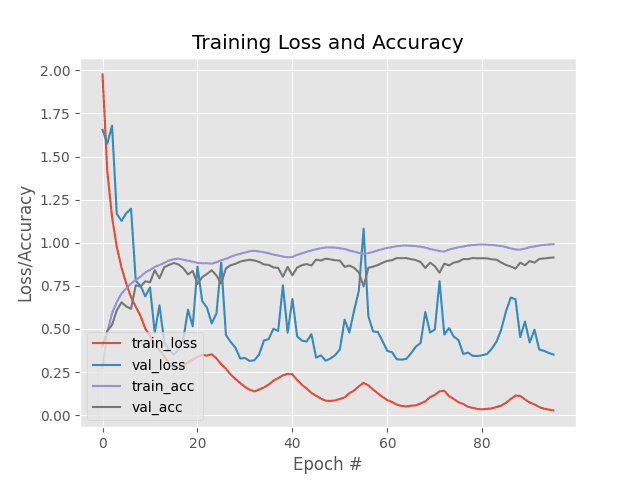Figure 8: Our first experiment training history plot shows the effects of the “triangular” policy on the accuracy/loss curves.

Notice the “wave” in the training accuracy and validation accuracy — the bottom of the wave is our base learning rate, the top of the wave is the upper bound on the learning rate, and the bottom of the wave, just before the next one starts, is the lower learning rate.

Just for fun, go back to Line 14 of the config.py file and update the CLR_METHOD to be triangular2 instead of triangular:

From there, train the network:

This time we are obtaining 90% accuracy, slightly lower than using the “triangular” policy.

Our learning rate plot visualizes how our learning rate is cyclically updated: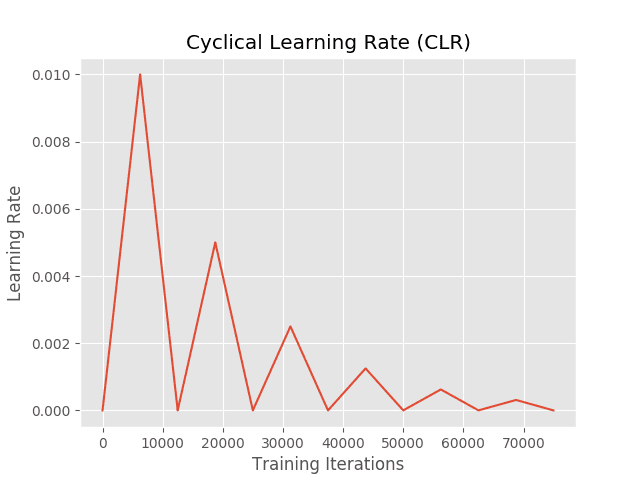Figure 9: Our second experiment uses the “triangular2” cyclical learning rate policy mode. The actual learning rates throughout training are shown in the plot.

Notice at after each complete cycle the maximum learning rate is halved. Since our maximum learning rate is decreasing after every cycle, our “waves” in the training and validation accuracy will be much less pronounced: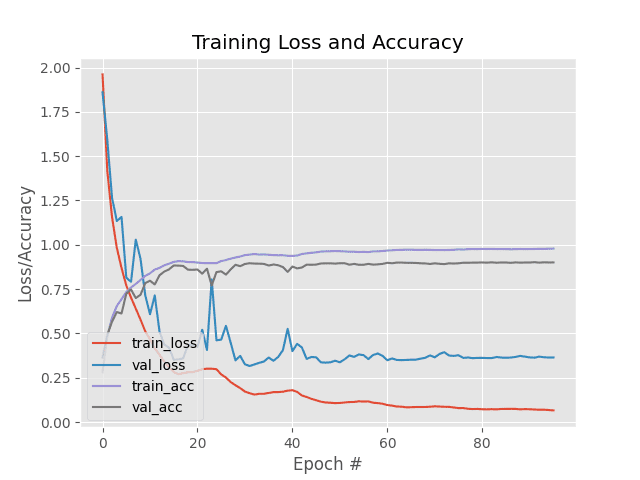Figure 10: Our second experiment training history plot shows the effects of the “triangular2” policy on the accuracy/loss curves.

While the “triangular” Cyclical Learning Rate policy obtained slightly better accuracy, it also exhibited far much fluctuation and had more risk of overfitting.

In contrast, the “triangular2” policy, while being less accurate, is more stable in its training.

When performing your own experiments with Cyclical Learning Rates I suggest you test both policies and choose the one that balances both accuracy and stability (i.e., stable training with less risk of overfitting).

In next week’s tutorial, I’ll show you how you can automatically define your minimum and maximum learning rate bounds with Cyclical Learning Rates.

If you’re interested in diving head-first into the world of computer vision/deep learning and discovering how to:

• Train Convolutional Neural Networks on your own custom datasets
• Replicate the results of state-of-the-art papers, including ResNet, SqueezeNet, VGGNet, and others
• Train your own custom Faster R-CNN, Single Shot Detectors (SSDs), and RetinaNet object detectors
• Train Generative Adversarial Networks (GANs)

…then be sure to take a look at my book, Deep Learning for Computer Vision with Python!

My complete, self-study deep learning book is trusted by members of top machine learning schools, companies, and organizations, including Microsoft, Google, Stanford, MIT, CMU, and more!

Readers of my book have gone on to win Kaggle competitions, secure academic grants, and start careers in CV and DL using the knowledge they gained through study and practice.

My book not only teaches the fundamentals, but also teaches advanced techniques, best practices, and tools to ensure that you are armed with practical knowledge and proven coding recipes to tackle nearly any computer vision and deep learning problem presented to you in school, in your research, or in the modern workforce.

Be sure to take a look  — and while you’re at it, don’t forget to grab your (free) table of contents + sample chapters.

## Summary

In this tutorial, you learned how to use Cyclical Learning Rates (CLRs) with Keras.

Unlike standard learning rate decay schedules, which monotonically decrease our learning rate, CLRs instead:

• Define a minimum learning rate
• Define a maximum learning rate
• Allow the learning rate to cyclically oscillate between the two bounds

Cyclical Learning rates often lead to faster convergence with fewer experiments and hyperparameter tuning.

But there’s still a problem…

How do we know that the optimal lower and upper bounds of the learning rate are?

That’s a great question — and I’ll be answering it in next week’s post where I’ll show you how to automatically find optimal learning rate values.

I hope you enjoyed today’s post!If you would like to download the code and images used in this post, please enter your email address in the form below. Not only will you get a .zip of the code, I’ll also send you a FREE 17-page Resource Guide on Computer Vision, OpenCV, and Deep Learning. Inside you'll find my hand-picked tutorials, books, courses, and libraries to help you master CV and DL! Sound good? If so, enter your email address and I’ll send you the code immediately!

### 12 Responses to Cyclical Learning Rates with Keras and Deep Learning

1.David Bonn July 29, 2019 at 7:55 pm #

Great and very helpful post as always. Do you have any insight into why CLR has not been included in Keras?

•Adrian Rosebrock August 6, 2019 at 12:42 pm #

I did some digging and actually found it in the keras-contrib package.

To quote their documentation:

“This library is the official extension repository for the python deep learning library Keras. It contains additional layers, activations, loss functions, optimizers, etc. which are not yet available within Keras itself. All of these additional modules can be used in conjunction with core Keras models and modules.

As the community contributions in Keras-Contrib are tested, used, validated, and their utility proven, they may be integrated into the Keras core repository. In the interest of keeping Keras succinct, clean, and powerfully simple, only the most useful contributions make it into Keras. This contribution repository is both the proving ground for new functionality, and the archive for functionality that (while useful) may not fit well into the Keras paradigm.”

I’m not sure why CLR wouldn’t be included in the primary package. If there is enough interest I imagine the group would merge it into the core package.

2.Aniket Saxena August 6, 2019 at 11:58 am #

Very good explanation and implementation details has been shown in this tutorial. Thanks for writing this tutorial.

•Adrian Rosebrock August 6, 2019 at 12:41 pm #

Thanks Aniket, I’m glad you liked it!

3.ali_beni August 7, 2019 at 3:11 pm #

A great and to the point tutorial. I have learned so much so far. So excited to read the next blog post about the automated LR scheduling. thank you and keep up the good work.

•Adrian Rosebrock August 16, 2019 at 6:06 am #

Thanks, I’m glad you enjoyed the guide!

4.Xu Zhang August 15, 2019 at 8:30 pm #

Is it only good for SGD optimizer? May I use it when I choose Adam as my optimizer? Many thanks.

•Adrian Rosebrock August 16, 2019 at 5:23 am #

You can use it with SGD, Adam, RMSprop, and other optimizers as well.

5.hosseinjan abaszadeh August 31, 2019 at 3:50 am #

firstly thanks for this great tutorial.
the most important problem is “LR range test”. This test is enormously valuable whenever you are facing a new architecture or dataset.
I am training a regressor network and this test is essential for my research to define base and max lr.
but i dont know how to do it. you said that:
“In next week’s tutorial, I’ll show you how you can automatically define your minimum and maximum learning rate bounds with Cyclical Learning Rates.”
have you posted this toturial?
if no, please put this toturial.
good Luck.

•Adrian Rosebrock September 5, 2019 at 10:01 am #

Yes, it’s been posted. You can find it here.

6.Ousama Esbel October 21, 2019 at 11:03 am #

Thank you for your great tutorial on how to use the CyclicLR, I learned alot from it. I only have one observation that hopefully you can help me out with:

Why did you start the optimizer with `base LR` and increase to `maximum LR` instead of starting with max LR and decrease to base LR? I think the later is better because you start with bigger LR and try to converge faster than starting from lower one wait a half cycle to build up speed.

•Adrian Rosebrock October 25, 2019 at 10:11 am #

You can think of the lower initial learning rate as a “warmup” period. For some models and datasets it’s been shown that a warmup period improves accuracy.